# Texas Go Math Grade 4 Unit 1 Answer Key Number and Operations: Place Value, Fraction Concepts, and Operations

Refer to our Texas Go Math Grade 4 Answer Key Pdf to score good marks in the exams. Test yourself by practicing the problems from Texas Go Math Grade 4 Unit 1 Answer Key Number and Operations: Place Value, Fraction Concepts, and Operations.

## Texas Go Math Grade 4 Unit 1 Answer Key Number and Operations: Place Value, Fraction Concepts, and Operations

Show What You Know

Read and Write Numbers Within 1,000

Write the number in different ways.

Question 1.The given figure is:From the given figure,
We can observe that
There are a total of 27 blocks
Now,
We know that,
“Expanded form” means writing the number so you show the value of each digit.
“Word form” means writing the number with words instead of numerals.
“Standard form” is a way of writing down very large or very small numbers easily
Hence, from the above,
We can conclude that
The representation of 27 in the following forms is:
Standard form: 27
Word form: Twenty-Seven
Expanded form: 20 + 7

Question 2.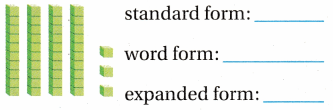The given figure is:From the given figure,
We can observe that
There are a total of 43 blocks
Now,
We know that,
“Expanded form” means writing the number so you show the value of each digit.
“Word form” means writing the number with words instead of numerals.
“Standard form” is a way of writing down very large or very small numbers easily
Hence, from the above,
We can conclude that
The representation of 43 in the following forms is:
Standard form: 43
Word form: Forty-Three
Expanded form: 40 + 3

Fractions of a Whole

Write a fraction that names the shaded part.

Question 3.The given model is: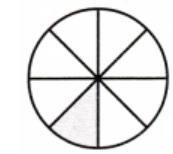Now,
From the above model,
We can observe that
The total number of parts are: 8
The number of shaded parts is: 1
Hence, from the above,
We can conclude that
The representation of the fraction that names the shaded part is: $$\frac{1}{8}$$

Question 4.The given model is: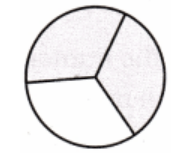Now,
From the above model,
We can observe that
The total number of parts are: 3
The number of shaded parts is: 2
Hence, from the above,
We can conclude that
The representation of the fraction that names the shaded part is: $$\frac{2}{3}$$

Question 5.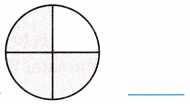The given model is: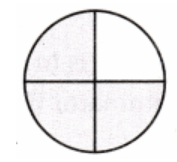Now,
From the above model,
We can observe that
The total number of parts are: 4
The number of shaded parts is: 3
Hence, from the above,
We can conclude that
The representation of the fraction that names the shaded part is: $$\frac{3}{4}$$

Question 6.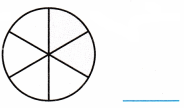The given model is: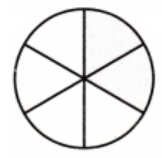Now,
From the above model,
We can observe that
The total number of parts are: 6
The number of shaded parts is: 2
Hence, from the above,
We can conclude that
The representation of the fraction that names the shaded part is: $$\frac{2}{6}$$

Question 7.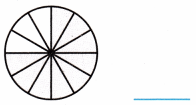The given model is:Now,
From the above model,
We can observe that
The total number of parts are: 12
The number of shaded parts is: 6
Hence, from the above,
We can conclude that
The representation of the fraction that names the shaded part is: $$\frac{6}{12}$$

Question 8.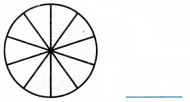The given model is: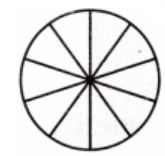Now,
From the above model,
We can observe that
The total number of parts are: 10
The number of shaded parts is: 2
Hence, from the above,
We can conclude that
The representation of the fraction that names the shaded part is: $$\frac{2}{10}$$

Fractions on a Number Line

Complete the number line. Draw a point to show the fraction.

Question 9.
$$\frac{4}{5}$$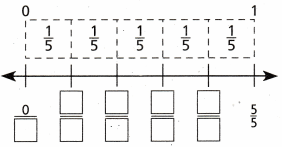The given fraction is: $$\frac{4}{5}$$
Now,
The representation of the complete number line is: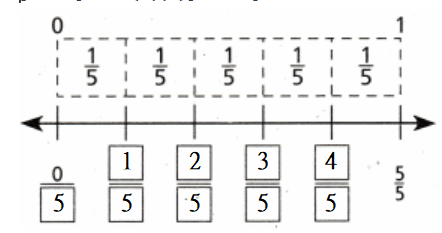Hence, from the above,
We can conclude that
The representation of the given fraction on the given number line is: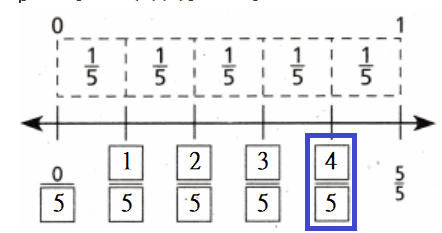Question 10.
$$\frac{3}{8}$$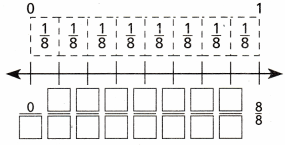The given fraction is: $$\frac{3}{8}$$
Now,
The representation of the complete number line is:Hence, from the above,
We can conclude that
The representation of the given fraction on the given number line is:Vocabulary Builder

Visualize It

Complete the flow map by using the words with a ✓.

Numbers and Operations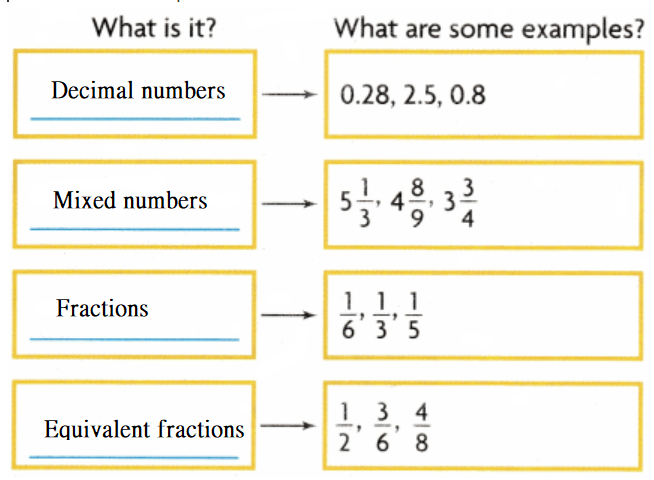Understand Vocabulary

Complete the sentences by using preview words.

Question 1.
A fraction is in ____________ if the numerator and denominator have only 1 as a common factor.
We know that,
A fraction is in “Simplest form” if the numerator and denominator have only 1 as a common factor.
Hence, from the above,
We can conclude that
The term that is suitable for the given statement is: Simplest form

Question 2.
A number close to an exact amount is an _____________ .
We know that,
A number close to an exact amount is an “Estimate” (or) “Approximate number”
Hence, from the above,
We can conclude that
The term that is suitable for the given statement is: Estimate (or) Approximate number

Question 3.
________________ is a way to write a number by showing the value of each digit.
We know that,
“Expanded form” is a way to write a number by showing the value of each digit.
Hence, from the above,
We can conclude that
The term that is suitable for the given statement is: Expanded form

Question 4.
A ________________ is a known size or amount that helps you understand a different size or amount.
We know that,
A “Benchmark” is a known size or amount that helps you understand a different size or amount.
Hence, from the above,
We can conclude that
The term that is suitable for the given statement is: Benchmark

Question 5.
A ________________ is one of ten equal parts.
We know that,
A “Tenth” is one of ten equal parts.
Hence, from the above,
We can conclude that
The term that is suitable for the given statement is: Tenth

Question 6.
A ________________ is one of one hundred equal parts.
We know that,
A “Hundredth” is one of one hundred equal parts.
Hence, from the above,
We can conclude that
The term that is suitable for the given statement is: Hundredth

Vocabulary

Look at the number in the place-value chart. Use this number to complete the word web.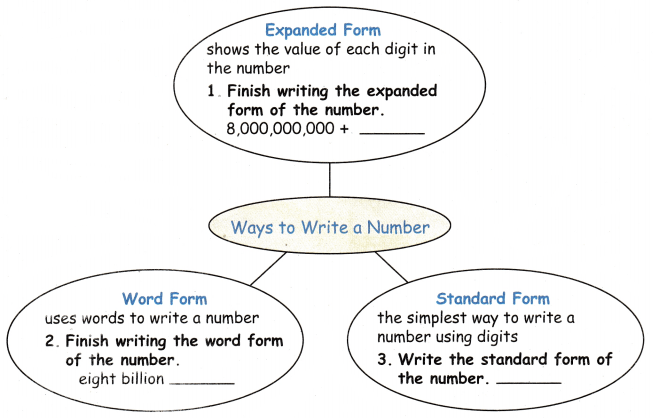The given number is: 8,827,610,731
Now,
The given place value chart for the given number is:Now,
We know that,
“Expanded form” means writing the number so you show the value of each digit.
“Word form” means writing the number with words instead of numerals.
“Standard form” is a way of writing down very large or very small numbers easily
Hence, from the above,
We can conclude that
The complete word web for the given place value chart is:
Expanded form:
8,000,000,000 + 800,000,000 + 20,000,000 + 7,000,000 + 600,000 + 10,000 + 0 + 700 + 30 + 1
Word form:
Eight billion Eight hundred twenty-seven million Six hundred ten thousand seven hundred thirty-one
Standard form:
8,827,610,731

Writing How can you write the 7536,301398 in word form?
The given number is: 7536,301398
Now,
We know that,
“Word form” means writing the number with words instead of numerals.
Hence, from the above,
We can conclude that
The representation of the word form for the given number is:
Seven billion Five hundred thirty-six million three hundred one thousand three hundred ninety-eight

Reading Look for this book in your library. Digging for Bird Dinosaurs: An Expedition to Madagascar, by Nic Bishop.

How to Play

Question 1.
Player 1 draws a card from the stack. He or she makes the fraction with the fraction strips and places them under the 1 whole strip.

Question 2.
Player 2 draws a card from the stack. He or she makes the fraction with the fraction strips and places them under Player 1’s strips.